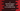# C program to find the largest two numbers in a number array### C program to find the largest two numbers in an array :

In this tutorial, we will learn how to find the largest two numbers in a given array using the C programming language. The program will ask the user how many numbers the array will contain and the user will give us the input of each number. Our program will then find out the largest and second-largest numbers in the array. Let’s take a look into the C program first. After that we will explain to you how the program works :

### C Program :

``````#include <stdio.h>
int main()
{
//1
int size;
int i;
//2
int largest = -1;
int secondLargest = -1;
//3
printf("How many elements you want to enter : ");
scanf("%d",&size);
//4
int array[size];
//5
for(i=0; i < size; i++){
printf("Enter : ");
//6
scanf("%d", &array[i]);
}

//7
for(i=0; i<size; i++){
//8
if(array[i] > largest){
secondLargest = largest;
largest = array[i];
}else if(array[i] > secondLargest){
//9
secondLargest = array[i];
}
}
//10
printf("First largest number is %d\n",largest);
printf("Second largest number is %d\n",secondLargest);
}``````

### Explanation :

The commented numbers in the above program denote the step number below :

1. Create one integer variable size to store the size of the array. Variable i is used for loops.
2. Create two variables to store largest and second largest numbers. These variables are largest and secondLargest. Both variables are assigned value -1.
3. Ask the user how many numbers he wants to enter. Read the value and store it in variable size.
4. Create one integer array of size equal to user input size.
5. Use one for loop to get the numbers for the array.
6. Ask the user to enter number and store it in the array. Get input of all numbers.
7. Run one more loop and read all numbers one by one.
8. If the current number is more than the largest number, assign value of largest to second largest and then assign that number to the largest.
9. If the current number is less than the largest but greater than the second largest, assign that number to the second largest number.
10. After the loop is completed, print the largest and second largest numbers.

### Sample Output :

``````How many elements you want to enter : 6
Enter number 1 : 12
Enter number 2 : 19
Enter number 3 : 90
Enter number 4 : 33
Enter number 5 : 10
Enter number 6 : 123
First largest number is 123 Second largest number is 90

How many elements you want to enter : 10
Enter number 1 : 1
Enter number 2 : 13
Enter number 3 : 15
Enter number 4 : 55
Enter number 5 : 43
Enter number 6 : 32
Enter number 7 : 21
Enter number 8 : 0
Enter number 9 : 122
Enter number 10 : 1000
First largest number is 1000 Second largest number is 122``````